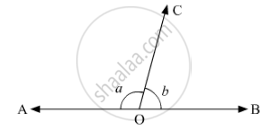# In Fig. 8.42, a is Greater than B by One Third of a Right-angle. Find the Values of a and B. - Mathematics

In Fig. 8.42, a is greater than b by one third of a right-angle. Find the values of a and b.#### Solution

Since a, b are linear pair

⇒ a + b = 180°

a = 180 - b                ........(1)

Now,

a = b + 1/3 × 90°                           [given]

⇒ a = b + 30°       .......(2)

⇒ a - b = 30°

Equating (1) and (2) equations

180 - b = b + 30°

⇒ 180° - 30° = b + b

⇒ 150° = 2b

⇒ b = (150°)/2

⇒ b = 75°

Hence a = 180 - b

= 180 - 75°             [ ∵ b = 75°]

a = 105°

∴ a = 105°, b = 75°

[  b = 75°]

Concept: Pairs of Angles
Is there an error in this question or solution?

#### APPEARS IN

RD Sharma Mathematics for Class 9
Chapter 10 Lines and Angles
Exercise 10.2 | Q 17 | Page 16